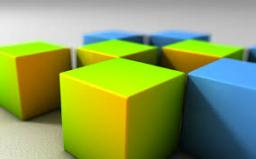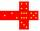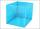# Cube 5

The content area of one cube wall is 32 square centimeters. Determine the length of its edges, its surface and volume.

a =  5.6569 cm
S =  192 cm2
V =  181.0193 cm3

### Step-by-step explanation:Did you find an error or inaccuracy? Feel free to write us. Thank you!Tips to related online calculators
Tip: Our volume units converter will help you with the conversion of volume units.

#### You need to know the following knowledge to solve this word math problem:

We encourage you to watch this tutorial video on this math problem:

## Related math problems and questions:

• The cubeThe surface of the cube is 150 square centimeters. Calculate: a- the content of its walls b - the length of its edges
• Cube wallCalculate the cube's diagonal if you know that one wall's surface is equal to 36 centimeters square. Please also calculate its volume.
• The cubeThe cube has a surface area of 216 dm2. Calculate: a) the content of one wall, b) edge length, c) cube volume.
• Cube wallThe surface of the first cube wall is 64 m2. The second cube area is 40% of the surface of the first cube. Determine the length of the edge of the second cube (x).
• Cuboid - VabFind the surface of the cuboid when its volume is 52.8 cubic centimeters, and the length of its two edges is 2 centimeters and 6 centimeters.
• Cube edgeDetermine the edges of the cube when the surface is equal to 37.5 cm square.
• Cube 5The surface of the cube is 15.36 dm2. How will change the surface area of this cube if the length of the edges is reduced by 2 cm?
• CubeThe sum of all cube edges is 30cm. Find the surface area of the cube.
• Cube edgesThe sum of the lengths of the cube edges is 42 cm. Calculate the surface of the cube.
• Cube V2SThe volume of the cube is 27 dm cubic. Calculate the surface of the cube.
• Cube 1-2-3Calculate the volume and surface area of the cube ABCDEFGH if: a) /AB/ = 4 cm b) perimeter of wall ABCD is 22 cm c) the sum of the lengths of all edges of the cube is 30 cm.
• The cubeThe cube has a surface area of 486 m ^ 2. Calculate its volume.
• TerezaThe cube has an area of base 256 mm2. Calculate the edge length, volume, and area of its surface.
• The cubeThe cube has a surface of 600 cm2. What is its volume?
• Cube wallsFind the volume and surface area of the cube if the area of one wall is 40cm2.
• Sum of the edgesThe sum of the lengths of all edges of the cube is 72 cm. How many cm2 of colored paper are we going to use for sticking?
• Cube wallThe perimeter of one cube wall is 120 meters. Calculate the surface area and the body diagonal of this cube.